• torch.topk(input, k, dim=None, largest=True, sorted=True, out=None) -> (Tensor, LongTensor) input：一个tensor数据 k：指明是得到前k个数据以及其index dim： 指定在哪个维度上排序， 默认是最后一个维度 ...
• 主要介绍了Java实现TopK问题的方法，文中通过示例代码介绍的非常详细，对大家的学习或者工作具有一定的参考学习价值，需要的朋友们下面随着小编来一起学习学习吧Java实现TopK
•top
• Tom Zu-search-topK-节省空间 链表实现的Top-K空间节省算法 2021/3 / 4-2021 / 3/5期间完成的工作 论文“数据流中频繁和Top-k元素的有效计算”中提出的算法 链接： ： 运行程序 将所有数据放入名称为fname的.txt...C++
• pytorch-topk.py

2020-12-25 11:52:06
以MNIST数据集为例，对LSTM的权重矩阵实现top-k剪枝（7，2），介绍了如何在pytorch框架下实现top-k剪枝。详细讲解参考这篇博客：https://blog.csdn.net/kuan__/article/details/111665163
• 主要介绍了使用堆实现Top K算法，即JS实现，文中详细介绍了Top K算法，感兴趣的小伙伴们可以参考一下
• TopK优化思路

2018-09-20 20:00:18
TopK，不难；其思路优化过程，不简单： • 全局排序，O(n*lg(n)) • 局部排序，只排序TopK个数，O(n*k) • 堆，TopK个数也不排序了，O(n*lg(k)) • 分治法，每个分支“都要”递归，例如：快速排序，O(n*lg(n)) • 减...算法
• topk 问题，就是从一个数组或者列表中获取最大的K个数，求3个积分，因为我需要3个积分下载东西，但是，我的里面的topK 解决方案肯定是比较全的，如果有什么看不懂的，请联系我，绝对负责给你讲清楚topK
• U-Topk是基于不确定性数据可能世界模型而提出的一种查询语义.随着不确定性数据集的增大,可能世界的实例数量指数增长,这为U-Topk查询处理提出了重大挑战.针对属性级不确定性的U-Topk查询处理算法展开研究,提出了U-Top...
• 基于二分查找的有序表，在做topK算法的给力实现
• TopK问题算法详解

千次阅读 2019-08-09 14:22:26
面试中，TopK，是问得比较多的几个问题之一，到底有几种方法，这些方案里蕴含的优化思路究竟是怎么样的，今天和大家聊一聊。 画外音：除非校招，我在面试过程中从不问TopK这个问题，默认大家都知道。 问题描述：...

内容

一、排序

二、局部排序

三、堆

四、随机选择

为各个算法添加了C++ 实现代码

面试中，TopK，是问得比较多的几个问题之一，到底有几种方法，这些方案里蕴含的优化思路究竟是怎么样的，今天和大家聊一聊。

画外音：除非校招，我在面试过程中从不问TopK这个问题，默认大家都知道。

问题描述：

从arr[1, n]这n个数中，找出最大的k个数，这就是经典的TopK问题。

栗子：

从arr[1, 12]={5,3,7,1,8,2,9,4,7,2,6,6} 这n=12个数中，找出最大的k=5个。

一、排序

排序是最容易想到的方法，将n个数排序之后，取出最大的k个，即为所得。

伪代码：

sort(arr, 1, n);

return arr[1, k];

C++ 代码:

/**
* 方法1 排序后取前K个数字 时间复杂度O(n*log(n)) 运行时间：4ms 占用内存：504k
* @param input vector
* @param k int
* @return
*/
vector<int> GetLeastNumbers_Solution1(vector<int> input, int k) {
vector<int> ans;
if (k > input.size() || input.size() == 0 || k == 0) {
return ans;
}
sort(input.begin(), input.end());
for (int i = 0; i < k; i++) {
ans.push_back(input[i]);
}
return ans;
}

时间复杂度：O(n*lg(n))

分析：明明只需要TopK，却将全局都排序了，这也是这个方法复杂度非常高的原因。那能不能不全局排序，而只局部排序呢？这就引出了第二个优化方法。

二、局部排序

不再全局排序，只对最大的k个排序。

冒泡是一个很常见的排序方法，每冒一个泡，找出最大值，冒k个泡，就得到TopK。

伪代码：

for(i=1 to k){

bubble_find_max(arr,i);

}

return arr[1, k];

C++代码:

/**
* 方法2 冒泡法, 每次取一个最小值, 时间复杂度O(n*k) 运行时间：4ms占用内存：468k
* @param input
* @param k
* @return
*/
vector<int> GetLeastNumbers_Solution2(vector<int> input, int k) {
vector<int> ans;
if (k > input.size() || input.size() == 0 || k == 0) {
return ans;
}
BubbleSort(input, k);
for (int i = 0; i < k; i++) {
ans.push_back(input[i]);
}
return ans;
}
/**
* 冒泡排序算法，只得到前ｋ个最小值
* @param input
* @param k
*/
void BubbleSort(vector<int> &input, int k) {
for (int i = 0; i < k; i++) {
int temp_i = i;
int count = input.size() - 1;
while (count - 1 >= temp_i) {
if (input[count] < input[count - 1]) {
int t = input[count - 1];
input[count - 1] = input[count];
input[count] = t;
}
count -= 1;
}
}
}

时间复杂度：O(n*k)

分析：冒泡，将全局排序优化为了局部排序，非TopK的元素是不需要排序的，节省了计算资源。不少朋友会想到，需求是TopK，是不是这最大的k个元素也不需要排序呢？这就引出了第三个优化方法。

三、堆

思路：只找到TopK，不排序TopK。

先用前k个元素生成一个小顶堆，这个小顶堆用于存储，当前最大的k个元素。

接着，从第k+1个元素开始扫描，和堆顶（堆中最小的元素）比较，如果被扫描的元素大于堆顶，则替换堆顶的元素，并调整堆，以保证堆内的k个元素，总是当前最大的k个元素。

直到，扫描完所有n-k个元素，最终堆中的k个元素，就是猥琐求的TopK。

伪代码：

heap[k] = make_heap(arr[1, k]);

for(i=k+1 to n){

}

return heap[k];

C++ 代码:

/**
* 建立最大堆
* @param input
* @param heap
* @param k　heap size
*/
void BuildMaxHeap(vector<int> &input, vector<int> &heap, int k) {
for (int i = 0; i < k; i++) {
heap.push_back(input[i]);
}
sort(heap.begin(), heap.end());
reverse(heap.begin(), heap.end());
}

/**
* 把heap堆顶的值下沉到合适的位置
* @param heap
*/
void ShiftMaxDown(vector<int> &heap) {
int cur_root = 0;
int cur_left = 2 * cur_root + 1;
int cur_right = 2 * cur_root + 2;
while (true) {
if (cur_left < heap.size() && heap[cur_root] < heap[cur_left]) {
int temp = heap[cur_root];
heap[cur_root] = heap[cur_left];
heap[cur_left] = temp;
cur_root = cur_left;
cur_left = 2 * cur_root + 1;
cur_right = 2 * cur_root + 2;
} else if (cur_right < heap.size() && heap[cur_root] < heap[cur_right]) {
int temp = heap[cur_root];
heap[cur_root] = heap[cur_right];
heap[cur_right] = temp;
cur_root = cur_right;
cur_left = 2 * cur_root + 1;
cur_right = 2 * cur_root + 2;
} else {
break;
}
}
}
/**
* 方法3 维护一个大小为K的最大堆(如果是求最大的K个数,则为最小堆), 时间复杂度O(n*log(k)), 运行时间：3ms占用内存：480k
* 该方法可以处理规模更大的数据（但是需要Ｋ较小，会有比较高的效率）,比如在内存中放不下,只能通过硬盘顺序读取.
* @param input
* @param k
* @return
*/
vector<int> GetLeastNumbers_Solution3(vector<int> input, int k) {
vector<int> ans;
if (k > input.size() || input.size() == 0 || k == 0) {
return ans;
}
BuildMaxHeap(input, ans, k);
for (int i = k; i < input.size(); i++) {
if (ans > input[i]) {
ans = input[i];
ShiftMaxDown(ans);
}
}
return ans;
}

时间复杂度：O(n*lg(k))

画外音：n个元素扫一遍，假设运气很差，每次都入堆调整，调整时间复杂度为堆的高度，即lg(k)，故整体时间复杂度是n*lg(k)。

分析：堆，将冒泡的TopK排序优化为了TopK不排序，节省了计算资源。堆，是求TopK的经典算法，那还有没有更快的方案呢？

四、随机选择

随机选择算在是《算法导论》中一个经典的算法，其时间复杂度为O(n)，是一个线性复杂度的方法。

这个方法并不是所有同学都知道，为了将算法讲透，先聊一些前序知识，一个所有程序员都应该烂熟于胸的经典算法：快速排序。

画外音：

（1）如果有朋友说，“不知道快速排序，也不妨碍我写业务代码呀”…额...

（2）除非校招，我在面试过程中从不问快速排序，默认所有工程师都知道；

其伪代码是：

void quick_sort(int[]arr, int low, inthigh){

if(low== high) return;

int i = partition(arr, low, high);

quick_sort(arr, low, i-1);

quick_sort(arr, i+1, high);

}

其核心算法思想是，分治法。

分治法（Divide&Conquer），把一个大的问题，转化为若干个子问题（Divide），每个子问题“都”解决，大的问题便随之解决（Conquer）。这里的关键词是“都”。从伪代码里可以看到，快速排序递归时，先通过partition把数组分隔为两个部分，两个部分“都”要再次递归。

分治法有一个特例，叫减治法。

减治法（Reduce&Conquer），把一个大的问题，转化为若干个子问题（Reduce），这些子问题中“只”解决一个，大的问题便随之解决（Conquer）。这里的关键词是“只”。

二分查找binary_search，BS，是一个典型的运用减治法思想的算法，其伪代码是：

int BS(int[]arr, int low, inthigh, int target){

if(low> high) return -1;

mid= (low+high)/2;

if(arr[mid]== target) return mid;

if(arr[mid]> target)

return BS(arr, low, mid-1, target);

else

return BS(arr, mid+1, high, target);

}

从伪代码可以看到，二分查找，一个大的问题，可以用一个mid元素，分成左半区，右半区两个子问题。而左右两个子问题，只需要解决其中一个，递归一次，就能够解决二分查找全局的问题。

通过分治法与减治法的描述，可以发现，分治法的复杂度一般来说是大于减治法的：

快速排序：O(n*lg(n))

二分查找：O(lg(n))

话题收回来，快速排序的核心是：

i = partition(arr, low, high);

这个partition是干嘛的呢？

顾名思义，partition会把整体分为两个部分。

更具体的，会用数组arr中的一个元素（默认是第一个元素t=arr[low]）为划分依据，将数据arr[low, high]划分成左右两个子数组：

左半部分，都比t大

右半部分，都比t小

中间位置i是划分元素

以上述TopK的数组为例，先用第一个元素t=arr[low]为划分依据，扫描一遍数组，把数组分成了两个半区：

左半区比t大

右半区比t小

中间是t

partition返回的是t最终的位置i。

很容易知道，partition的时间复杂度是O(n)。

画外音：把整个数组扫一遍，比t大的放左边，比t小的放右边，最后t放在中间N[i]。

partition和TopK问题有什么关系呢？

TopK是希望求出arr[1,n]中最大的k个数，那如果找到了第k大的数，做一次partition，不就一次性找到最大的k个数了么？

画外音：即partition后左半区的k个数。

问题变成了arr[1, n]中找到第k大的数。

再回过头来看看第一次partition，划分之后：

i = partition(arr, 1, n);

如果i大于k，则说明arr[i]左边的元素都大于k，于是只递归arr[1, i-1]里第k大的元素即可；

如果i小于k，则说明说明第k大的元素在arr[i]的右边，于是只递归arr[i+1, n]里第k-i大的元素即可；

画外音：这一段非常重要，多读几遍。

这就是随机选择算法randomized_select，RS，其伪代码如下：

int RS(arr, low, high, k){

if(low== high) return arr[low];

i= partition(arr, low, high);

temp= i-low; //数组前半部分元素个数

if(temp>=k)

return RS(arr, low, i-1, k); //求前半部分第k大

else

return RS(arr, i+1, high, k-i); //求后半部分第k-i大

}

C++ 代码:

/**
* quick sort core
* @param input
* @param start
* @param end
* @return
*/
int Partition(vector<int> &input, int start, int end) {
int temp = input[start];
while (start < end) {
while (start < end && temp <= input[end]) {
end -= 1;
}
input[start] = input[end];
if (start < end) {
start += 1;
while (start < end && temp > input[start]) {
start += 1;
}
input[end] = input[start];
end -= 1;
}
}
input[start] = temp;
return start;
}

/**
*方法4 基于快排的方法,一次定位到key之后,如果key的index + 1==K,那么这前K个刚好就是要求最小的值, 复杂度O(n), 运行时间：3ms 占用内存：480k
*如果key的index＋１> K则继续递归前半部分即可,如果key的index+1<K,只需要递归后半部分
* @param input
* @param k
* @return
*/
vector<int> GetLeastNumbers_Solution4(vector<int> input, int k) {
vector<int> ans;
if (k > input.size() || input.size() == 0 || k == 0) {
return ans;
}
int start = 0;
int end = input.size() - 1;
int part_index = Partition(input, 0, input.size() - 1);
while (part_index + 1 != k) {
if (part_index + 1 > k) {
end = part_index - 1;
part_index = Partition(input, start, end);
} else {
start = part_index + 1;
part_index = Partition(input, start, end);
}
}
for (int i = 0; i < k; i++) {
ans.push_back(input[i]);
}
return ans;
}

这是一个典型的减治算法，递归内的两个分支，最终只会执行一个，它的时间复杂度是O(n)。

再次强调一下：

分治法，大问题分解为小问题，小问题都要递归各个分支，例如：快速排序

减治法，大问题分解为小问题，小问题只要递归一个分支，例如：二分查找，随机选择

通过随机选择（randomized_select），找到arr[1, n]中第k大的数，再进行一次partition，就能得到TopK的结果。

五、总结

TopK，不难；其思路优化过程，不简单：

全局排序，O(n*lg(n))

局部排序，只排序TopK个数，O(n*k)

堆，TopK个数也不排序了，O(n*lg(k))

分治法，每个分支“都要”递归，例如：快速排序，O(n*lg(n))

减治法，“只要”递归一个分支，例如：二分查找O(lg(n))，随机选择O(n)

TopK的另一个解法：随机选择+partition

知其然，知其所以然。

思路比结论重要。

希望大家对TopK有新的认识，谢转。
原文链接：https://blog.csdn.net/z50L2O08e2u4afToR9A/article/details/82837278

展开全文• Topk 问题描述 如从海量数据中寻找最大(或最小)的 k 个元素，这类问题被称为 Topk问题。这个问题无论在实际应用还是面试都会被问到。那我们今天就来看看到底有几种解决方案，以及各个方案的优劣情况。以下解题思路...

Topk 问题描述

从海量数据中寻找最大(或最小)的 k 个元素，这类问题称为 Topk 问题。这个问题无论在实际应用还是面试都会被问到。那我们今天就来看看到底有几种解决方案，以及各个方案的优劣情况。以下解题思路的前提条件是：从数组array[1, n]中，寻找出最大的 k 个数。

全局排序

面对Topk问题，最容易想到的办法就是排序了。将array里的元素进行排列，便可以获得最大的 k 个数。此时，Topk问题就转变成了排序问题，解决Topk问题的时间复杂度变成了排序的时间复杂度。如果使用快排进行排序，那么该问题的时间复杂度就是O(n*lgn)。

局部排序

由于是寻找Topk，所以没有必要对所有的数据都进行排序。虽然快排的表现较好，但是如果使用冒泡或简单选择排序，只需要完成k次排序操作就可以解决问题，即如下图所示。此时的时间复杂度是O(n*k)。注意：局部排序所耗费的时间受 k 值影响。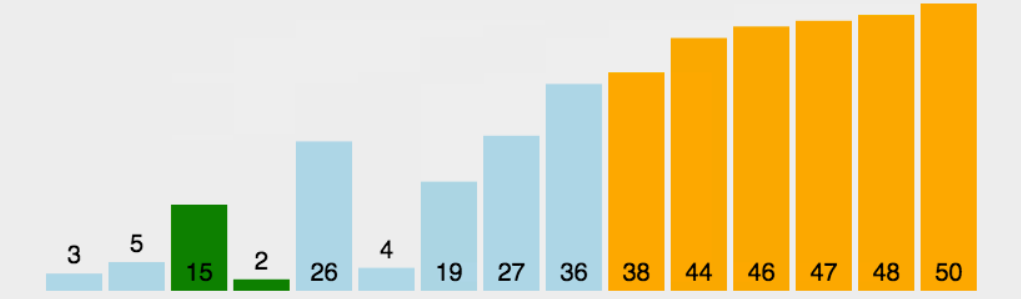思路：遍历整个数组，在遍历的过程中利用小根堆记录当前的Topk元素。因为小根堆的最小的元素在堆顶，如果下一个元素大于堆顶元素值，那么它就能入选当前的Topk。关于堆的概念和代码可以看看这篇文章：通俗易懂的讲解堆排序(含Gif图)

例如：从list[ n ] = {58, 32, 73, 20, 31, 95, 46, 77, 22, 67,..., n}中寻找最大的5个数。首先利用前5个元素建立堆，然后再与后续的元素进行对比，不断的和堆顶元素进行对比。若元素大于堆顶元素，则进行替换，然后调整堆，使其一直保持小根堆的性质。所有元素比对完成后，堆中的元素就是该序列中最大的5个数。

初始建堆：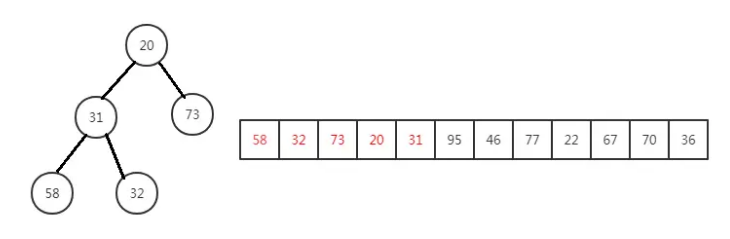使用95与堆顶元素对比，若大于堆顶元素，则替换：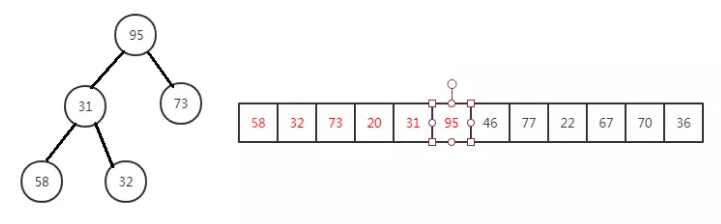替换95后需要调整堆：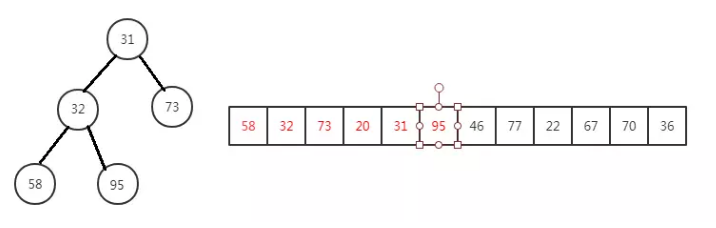重复上述操作，直至所有元素比对完成，堆中的元素就是所求的最大的 5个元素。

代码实现

#include <iostream>
#include <time.h>
#include <stdlib.h>
#include <cstdlib>
#include <stdio.h>
#include <math.h>
#define M 1000000
#define K 50
using namespace std;

template <class T>
void Print(T a[], int n, int m)
{
for(int i = n; i < m; i++)
{
cout << "[" << a[i] << "]";
}
cout <<endl;
}

template <class T>
void Swap(T &a, T &b)
{
T asd;
asd = a;
a = b;
b = asd;
}

template <class T>
int Partition(T a[], int p, int r)
{
int i = p, j = r+1;
T x = a[p];
while(true)
{
while(a[++i] < x && i < r);
while(a[--j] > x);
if(i >= j)break;
Swap(a[i], a[j]);
}
a[p] = a[j];
a[j] = x;
return j;
}

template <class T>
void QuickSort(T a[], int p, int r)
{
if(p < r)
{
int q = Partition(a, p, r);
QuickSort(a, p, q-1);
QuickSort(a, q+1, r);
}
}

void test(int a[])
{
int i,temp;
for(i = 0; i < M; ++i)
{
if(a[i] > i)
temp = 1;
else
temp = 0;
}
}

void BubbleSort(int a[])
{
int i,j,flag,temp;
for(i = 0; i < K; ++i)
{
flag = 0;
for(j = 0; j < M-i-1; ++j)
{
if(a[j] > a[j+1])
{
temp = a[j];
a[j] = a[j+1];
a[j+1] = temp;
flag = 1;
}
}
if(flag == 0) break;
}
}

void BubbleSort1(int a[], int n)
{
int i,j,flag,temp;
for(i = 0; i < n; ++i)
{
flag = 0;
for(j = 0; j < n-i-1; ++j)
{
if(a[j] > a[j+1])
{
temp = a[j];
a[j] = a[j+1];
a[j+1] = temp;
flag = 1;
}
}
if(flag == 0) break;
}
}

void heap_ajust_min(int *b, int i, int size)  /*a为堆存储数组，size为堆的大小*/
{
int lchild = 2*i;       //i的左孩子节点序号
int rchild = 2*i +1;     //i的右孩子节点序号
int min = i; /*存放三个顶点中最大的数的下标*/
int temp;
if(i <= size/2)          //假设i是叶节点就不用进行调整
{
if(lchild<=size && b[lchild]<b[min])
{
min = lchild;
}
if(rchild<=size && b[rchild]<b[min])
{
min = rchild;
}
if(min != i)
{
temp = b[i]; /*交换a[i]和a[max]的值*/
b[i] = b[min];
b[min] = temp;
heap_ajust_min(b, min, size); /*被交换的位置曾经是大根堆，如今可能不是大根堆
所以须要又一次调整使其成为大根堆结构*/
}
}
}

void build_bheap_min(int *b, int size) /*建立小堆*/
{
int i;
for(i=size/2; i >= 1; i--) /*非叶节点最大序号值为size/2*/
{
heap_ajust_min(b, i, size); /*每一个非叶子结点都须要调用这个函数*/
}
}

int a[M] = {0};
int a1[M] = {0};
int a2[M] = {0};
int a3[M] = {0};
int b[K+1]={0};
int c[K] = {0};

int main()
{
srand(time(0));

for(int i = 0; i < M; i++)
{
a[i] = rand()%(M);       //设置随机数组
a1[i] = rand()%(M);
a2[i] = rand()%(M);
a3[i] = rand()%(M);
}

//方法一
clock_t start_time = clock();
QuickSort(a, 0, M-1);
clock_t end_time = clock();
cout<<"快排全排列:"<<static_cast<double>(end_time - start_time)/CLOCKS_PER_SEC*1000<<"ms"<<endl;

//方法二
clock_t start_time1 = clock();
BubbleSort(a1);
clock_t end_time1 = clock();
cout<<"冒泡排列k次:"<<static_cast<double>(end_time1 - start_time1)/CLOCKS_PER_SEC*1000<<"ms"<<endl;

//方法三
clock_t start_time2 = clock();

for(int i = 0; i < M; ++i)
{
if(i < K)
{
c[i] = a2[i];
}
else
{
if(a2[i] > c)
{
c = a2[i];
BubbleSort1(c, K);
}
}
}

clock_t end_time2 = clock();
cout<<"使用冒泡方法记录Topk:"<<static_cast<double>(end_time2 - start_time2)/CLOCKS_PER_SEC*1000<<"ms"<<endl;

//方法四
clock_t start_time3 = clock();

for(int i = 0; i < M; ++i)
{
if(i <= K)
{
b[i+1] = a3[i];
}
else
{
if(a3[i] > b)
{
b = a3[i];
build_bheap_min(b, K);
}
}
}

clock_t end_time3 = clock();
cout<<"使用小根堆记录Topk:"<<static_cast<double>(end_time3 - start_time3)/CLOCKS_PER_SEC*1000<<"ms"<<endl;

return 0;
}

实验

在100万个元素的情况下，分别令 K 的值为10和50。实验结果如下：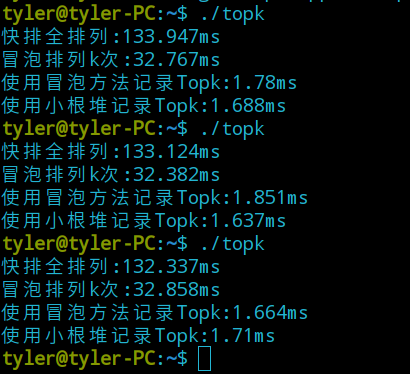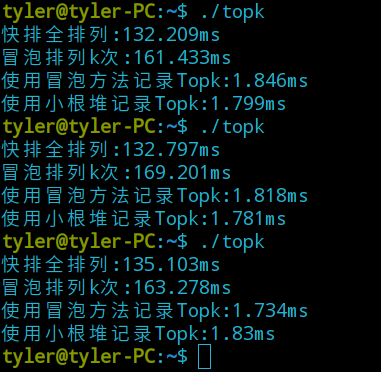可以发现冒泡排序受 K 的变化而变化，而其他的三种方式较为稳定。四种方法中表现较好的是后两种，为了比个高低，我设置了1000万个元素，分别令K值为1000和10000，实验结果如下：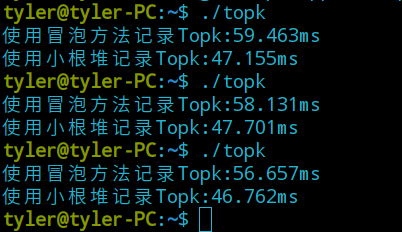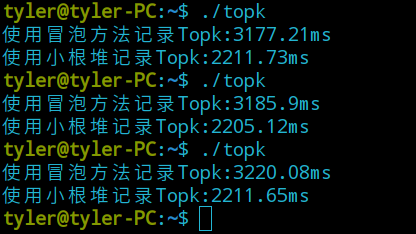在扩充了元素和改变 K 值后可发现，利用小根堆的方法性能更好。原因：假设在最坏的情况下，方法三每次完成一次排序，方法四每次都要调整堆。方法三的时间复杂度O(n*k)，而方法四的时间复杂度为O(n*lg(k))

展开全文Topk
• TopK算法分析

万次阅读 多人点赞 2018-10-04 20:35:33
TopK，是问得比较多的几个问题之一，到底有几种方法，这些方案里蕴含的优化思路究竟是怎么样的，今天和大家聊一聊。 问题描述： 从arr[1, n]这n个数中，找出最大的k个数，这就是经典的TopK问题。 栗子： 从arr[1...

个人博客请访问 http://www.x0100.top

TopK，是问得比较多的几个问题之一，到底有几种方法，这些方案里蕴含的优化思路究竟是怎么样的，今天和大家聊一聊。

问题描述

从arr[1, n]这n个数中，找出最大的k个数，这就是经典的TopK问题。

栗子

从arr[1, 12]={5,3,7,1,8,2,9,4,7,2,6,6} 这n=12个数中，找出最大的k=5个。

一、排序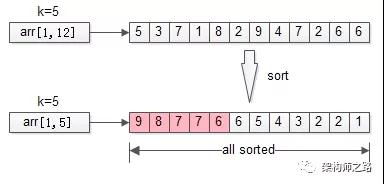排序是最容易想到的方法，将n个数排序之后，取出最大的k个，即为所得。

伪代码

sort(arr, 1, n);

return arr[1, k];

时间复杂度：O(n*lg(n))

分析：明明只需要TopK，却将全局都排序了，这也是这个方法复杂度非常高的原因。那能不能不全局排序，而只局部排序呢？这就引出了第二个优化方法。

二、局部排序

不再全局排序，只对最大的k个排序。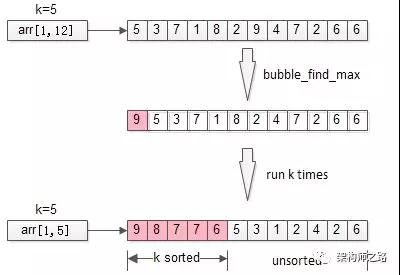冒泡是一个很常见的排序方法，每冒一个泡，找出最大值，冒k个泡，就得到TopK。

伪代码

for(i=1 to k){

bubble_find_max(arr,i);

}

return arr[1, k];

时间复杂度：O(n*k)

分析：冒泡，将全局排序优化为了局部排序，非TopK的元素是不需要排序的，节省了计算资源。不少朋友会想到，需求是TopK，是不是这最大的k个元素也不需要排序呢？这就引出了第三个优化方法。

三、堆

思路：只找到TopK，不排序TopK。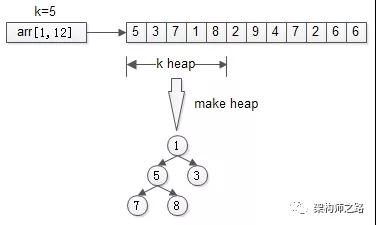先用前k个元素生成一个小顶堆，这个小顶堆用于存储，当前最大的k个元素。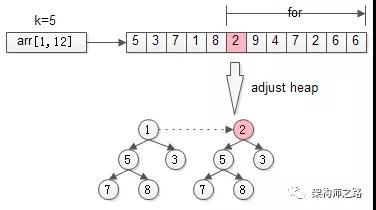接着，从第k+1个元素开始扫描，和堆顶（堆中最小的元素）比较，如果被扫描的元素大于堆顶，则替换堆顶的元素，并调整堆，以保证堆内的k个元素，总是当前最大的k个元素。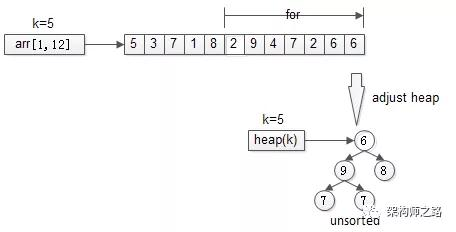直到，扫描完所有n-k个元素，最终堆中的k个元素，就是猥琐求的TopK。

伪代码

heap[k] = make_heap(arr[1, k]);

for(i=k+1 to n){

}

return heap[k];

时间复杂度：O(n*lg(k))

画外音：n个元素扫一遍，假设运气很差，每次都入堆调整，调整时间复杂度为堆的高度，即lg(k)，故整体时间复杂度是n*lg(k)。

分析：堆，将冒泡的TopK排序优化为了TopK不排序，节省了计算资源。堆，是求TopK的经典算法，那还有没有更快的方案呢？

四、随机选择

随机选择算在是《算法导论》中一个经典的算法，其时间复杂度为O(n)，是一个线性复杂度的方法。

这个方法并不是所有同学都知道，为了将算法讲透，先聊一些前序知识，一个所有程序员都应该烂熟于胸的经典算法：快速排序。

画外音：

（1）如果有朋友说，“不知道快速排序，也不妨碍我写业务代码呀”…额...

（2）除非校招，我在面试过程中从不问快速排序，默认所有工程师都知道；

其伪代码是

void quick_sort(int[]arr, int low, inthigh){

if(low== high) return;

int i = partition(arr, low, high);

quick_sort(arr, low, i-1);

quick_sort(arr, i+1, high);

}

其核心算法思想是，分治法。

分治法（Divide&Conquer），把一个大的问题，转化为若干个子问题（Divide），每个子问题“”解决，大的问题便随之解决（Conquer）。这里的关键词是“都”。从伪代码里可以看到，快速排序递归时，先通过partition把数组分隔为两个部分，两个部分“都”要再次递归。

分治法有一个特例，叫减治法。

减治法（Reduce&Conquer），把一个大的问题，转化为若干个子问题（Reduce），这些子问题中“”解决一个，大的问题便随之解决（Conquer）。这里的关键词是“只”

二分查找binary_search，BS，是一个典型的运用减治法思想的算法，其伪代码是：

int BS(int[]arr, int low, inthigh, int target){

if(low> high) return -1;

mid= (low+high)/2;

if(arr[mid]== target) return mid;

if(arr[mid]> target)

return BS(arr, low, mid-1, target);

else

return BS(arr, mid+1, high, target);

}

从伪代码可以看到，二分查找，一个大的问题，可以用一个mid元素，分成左半区，右半区两个子问题。而左右两个子问题，只需要解决其中一个，递归一次，就能够解决二分查找全局的问题。

通过分治法与减治法的描述，可以发现，分治法的复杂度一般来说是大于减治法的：

快速排序：O(n*lg(n))

二分查找：O(lg(n))

话题收回来，快速排序的核心是：

i = partition(arr, low, high);

这个partition是干嘛的呢？

顾名思义，partition会把整体分为两个部分。

更具体的，会用数组arr中的一个元素（默认是第一个元素t=arr[low]）为划分依据，将数据arr[low, high]划分成左右两个子数组：

• 左半部分，都比t大

• 右半部分，都比t小

• 中间位置i是划分元素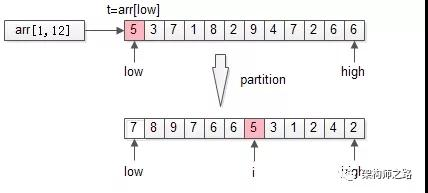以上述TopK的数组为例，先用第一个元素t=arr[low]为划分依据，扫描一遍数组，把数组分成了两个半区：

• 左半区比t大

• 右半区比t小

• 中间是t

partition返回的是t最终的位置i。

很容易知道，partition的时间复杂度是O(n)。

画外音：把整个数组扫一遍，比t大的放左边，比t小的放右边，最后t放在中间N[i]。

partition和TopK问题有什么关系呢？

TopK是希望求出arr[1,n]中最大的k个数，那如果找到了第k大的数，做一次partition，不就一次性找到最大的k个数了么？

画外音：即partition后左半区的k个数。

问题变成了arr[1, n]中找到第k大的数。

再回过头来看看第一次partition，划分之后：

i = partition(arr, 1, n);

• 如果i大于k，则说明arr[i]左边的元素都大于k，于是只递归arr[1, i-1]里第k大的元素即可；

• 如果i小于k，则说明说明第k大的元素在arr[i]的右边，于是只递归arr[i+1, n]里第k-i大的元素即可；

画外音：这一段非常重要，多读几遍。

这就是随机选择算法randomized_select，RS，其伪代码如下：

int RS(arr, low, high, k){

if(low== high) return arr[low];

i= partition(arr, low, high);

temp= i-low; //数组前半部分元素个数

if(temp>=k)

return RS(arr, low, i-1, k); //求前半部分第k大

else

return RS(arr, i+1, high, k-i); //求后半部分第k-i大

}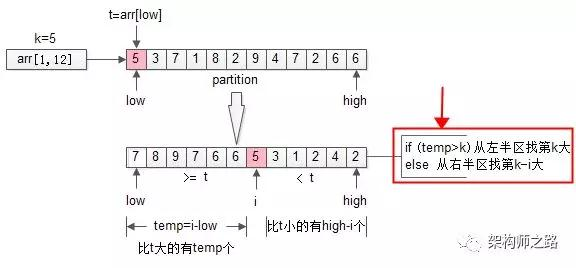这是一个典型的减治算法，递归内的两个分支，最终只会执行一个，它的时间复杂度是O(n)。

再次强调一下：

• 分治法，大问题分解为小问题，小问题都要递归各个分支，例如：快速排序

• 减治法，大问题分解为小问题，小问题只要递归一个分支，例如：二分查找，随机选择

通过随机选择（randomized_select），找到arr[1, n]中第k大的数，再进行一次partition，就能得到TopK的结果。

五、总结

TopK，不难；其思路优化过程，不简单：

• 全局排序，O(n*lg(n))

• 局部排序，只排序TopK个数，O(n*k)

• ，TopK个数也不排序了，O(n*lg(k))

• 分治法，每个分支“都要”递归，例如：快速排序，O(n*lg(n))

• 减治法，“只要”递归一个分支，例如：二分查找O(lg(n))，随机选择O(n)

• TopK的另一个解法：随机选择+partition

知其然，知其所以然。

思路比结论重要。

更多精彩内容扫描下方二维码进入网站。。。。。关注微信公众号。。。。。展开全文算法
• 大数据量获取TopK的几种方案

千次阅读 2018-09-30 14:27:03
生活中经常会遇到求TopK的问题，在小数据量的情况下可以先将所有数据排序，最后进行遍历。但是在大数据量情况下，这种的时间复杂度最低的也就是O(NlogN)此处的N可能为10亿这么大的数字，时间复杂度过高，那么什么...

一：介绍

生活中经常会遇到求TopK的问题，在小数据量的情况下可以先将所有数据排序，最后进行遍历。但是在大数据量情况下，这种的时间复杂度最低的也就是O(NlogN)此处的N可能为10亿这么大的数字，时间复杂度过高，那么什么方法可以减少时间复杂度呢，以下几种方式，与大家分享。

二：局部淘汰法 -- 借助“冒泡排序”获取TopK

1. 思路：

• 可以避免对所有数据进行排序，只排序部分

• 冒泡排序是每一轮排序都会获得一个最大值，则K轮排序即可获得TopK

2. 时间复杂度空间复杂度

3. 代码比较简单就不贴了，只要会写冒泡就ok了

三：局部淘汰法 -- 借助数据结构"堆"获取TopK

1. 思路：

• 堆：分为大顶堆（堆顶元素大于其他所有元素）和小顶堆（堆顶其他元素小于所有其他元素）

• 我们使用小顶堆来实现，为什么不适用大顶堆下面会介绍~

• 取出K个元素放在另外的数组中，对这K个元素进行建堆 ps:堆排序请参考：https://blog.csdn.net/CSDN___LYY/article/details/81454613

• 然后循环从K下标位置遍历数据，只要元素大于堆顶，我们就将堆顶赋值为该元素，然后重新调整为小顶堆

• 循环完毕后，K个元素的堆数组就是我们所需要的TopK

2. 为什么使用小顶堆呢？

• 我们在比较的过程中使用堆顶是最小值的小顶堆，元素大于堆顶我们对堆顶进行重新赋值，那么堆顶永远是这K个值中最小的值，当我们下一个元素和堆顶比较时，如果不大于堆顶的话，那么一定不属于topK范围的

3. 时间复杂度与空间复杂度

• 时间复杂度：每次对K个元素进行建堆，时间复杂度为：O(KlogK)，加上N-K次的循环，则总时间复杂度为O((K+(N-K))logK)，即O(NlogK)，其中K为想要获取的TopK的数量N为总数据量

• 空间复杂度：O(K)，只需要新建一个K大小的数组用来存储topK即可

4. 适用环境

• 适用于单核单机环境，不会发挥多核的优势

• 也可用于分治法中获取每一份元素的Top，下面会介绍

5. 代码实现

• 使用的java代码实现的，代码内每一步都有注释便于理解

import java.util.Arrays;

/**
* 通过堆这种数据结构
* 获得大数据量中的TopK
*/

public class TopKStack {
public static void main(String[] args) {
//定义一个数组，找出该数组中的topK，大数据量不好搞到，先用这个数组测试
int [] datas = {2,3,42,1,34,5,6,67,3,243,8,246,123,6,32,3451,23,5,6,31,5,6,2346,36};
int [] re = getTopK(datas,10);
System.out.println(Arrays.toString(re));
}

/**
* 获取前topk的方法
* @param datas 原数组
* @param num 前topNum
* @return 最后的topNum的堆数组
*/
static int[] getTopK(int[] datas,int num){
//定义存储前num个元素的数组，用于建堆
int[] res = new int[num];
//初始化数组
for (int i = 0; i < num; i++) {
res[i] = datas[i];
}
//建造初始化堆
for (int i = (num - 1)/2; i >= 0 ; i--) {
shift(res,i);
}
//遍历查找num个最大值
for (int i = num; i < datas.length; i++) {
if (datas[i] > res){
res = datas[i];
shift(res,0);
}
}
return res;
}

/**
* 调整元素满足堆结构
* @param datas
* @param index
* @return
*/
static int[] shift(int[] datas ,int index){
while(true){
int left = (index<<1) + 1; //左孩子
int right = (index<<1) + 2; //右孩子

int min_num = index; //标识自身节点和孩子节点中最小值的位置
//判断是否存在左右孩子，并且得到左右孩子和自身的最小值
if (left <= datas.length-1&&datas[left] < datas[index]){
min_num = left;
}
if (right <= datas.length-1&&datas[right] < datas[min_num]){
min_num = right;
}
//如果最小值不等于自身，则将最小值与自身交换
if (min_num != index){
int temp = datas[index];
datas[index] = datas[min_num];
datas[min_num] = temp;
}else{
//此处break是因为我们是从树的最下面进行调整的，如果上层节点符合堆，则下层节点一定符合！
break;
}

//执行到此处，说明可能需要调整下面的节点，则将初始节点赋值为最小值所在的节点位置，
// 因为最大值点的位置进行了交换，可能下层节点就不满足堆性质
index = min_num;
}
return datas;
}
}

四：分治法 -- 借助”快速排序“方法获取TopK

1. 思路：

• 比如有10亿的数据，找处Top1000，我们先将10亿的数据分成1000份，每份100万条数据

• 在每一份中找出对应的Top 1000，整合到一个数组中，得到100万条数据，这样过滤掉了999%%的数据

• 使用快速排序对这100万条数据进行”一轮“排序，一轮排序之后指针的位置指向的数字假设为S，会将数组分为两部分，一部分大于S记作Si，一部分小于S记作Sj。 ps:快速排序请参考：https://blog.csdn.net/CSDN___LYY/article/details/81478583

• 如果Si元素个数大于1000，我们对Si数组再进行一轮排序，再次将Si分成了Si和Sj。如果Si的元素小于1000，则我们需要在Sj中获取1000-count(Si)个元素的，也就是对Sj进行排序

• 如此递归下去即可获得TopK

2. 和第一种方法有什么不同呢？相对来说的优点是什么？

• 第二种方法中我们可以采用多核的优势，创建多个线程，分别去操作不同的数据。

• 当然我们在分治的第二步可以使用第一种方法去获取每一份的Top。

3. 适用环境

• 多核多机的情况，分治法会将多核的作用发挥到最大，节省大量时间

4. 时间复杂度与空间复杂度

• 时间复杂度：一份获取前TopK的时间复杂度：O((N/n)logK)。则所有份数为：O(NlogK)，但是分治法我们会使用多核多机的资源，比如我们有S个线程同时处理。则时间复杂度为：O((N/S)logK)。之后进行快排序，一次的时间复杂度为：O(N),假设排序了M次之后得到结果，则时间复杂度为：O(MN)。所以 ，总时间复杂度大约为O(MN+(N/S)logK) 。

• 空间复杂度：需要每一份一个数组，则空间复杂度为O(N)

五：其他情况

• 通常我们要根据数据的情况去判断我们使用什么方法，在获取TopK前我们可以做什么操作减少数据量。

• 比如：数据集中有许多重复的数据并且我们需要的是前TopK个不同的数，我们可以先进行去重之后再获取前TopK。如何进行大数据量的去重操作呢，简单的说一下：

1. 采用bitmap来进行去重。

2. 一个char类型的数据为一个字节也就是8个字符，而每个字符都是用0\1标识，我们初始化所有字符为0。

3. 我们申请N/8+1容量的char数组，总共有N+8个字符。

4. 对数据进行遍历，对每个元素S进行S/8操作获得char数组中的下标位置，S%8操作获得该char的第几个字符置1。

5. 在遍历过程中，如果发现对应的字符位置上已经为1,则代表该值为重复值，可以去除。

• 主要还是根据内存、核数、最大创建线程数来动态判断如何获取前TopK。

展开全文大数据量TopK TopK 算法
• TopK问题详解

千次阅读 2019-05-29 17:22:30
面试中经常会问到的一道题目：从n个未排序的数中得到的最大的k个数，称为TopK问题。（最小的k个数做法也相似） 基于partition函数 基于快速排序中的partition函数，时间复杂度为O（n），空间复杂度为O（1）；需要...topk
• torch.topk()函数快速理解

千次阅读 热门讨论 2021-08-23 22:05:22
该函数的作用即按字面意思理解，topk:取数组的前k个元素进行排序。 通常该函数返回2个值，第一个值为排序的数组，第二个值为该数组中获取到的元素在原数组中的位置标号。 举个栗子： import numpy as np import ...python pytorch
• topk 算法

2013-10-15 20:37:56
• topk算法实现

千次阅读 2019-03-01 11:07:27
top k就是求解一个数字队列前k大的问题，在工作后者面试中是一个非常常见的问题，这里说明两种解法。 1.基于快排的解法 1.1 算法思路 这里假设你对快排已经熟悉。我们知道快排是随机找个标识，然后用此标识进行排序...
• MapReduce实现TopK算法（实战）

千次阅读 2019-10-24 10:29:06
MapReduce实现TopK算法（实战） 项目介绍 数据集下载 程序下载 代码讲解 运行结果示例MapReduce TopK Hadoop Java 算法
• 此种方法就不多做解释了，就是使用快排，归并，堆排序等方法先将数组完全排序，然后再取topK，时间复杂度为O(NlogN)。而且这种方法不适用于大数据量，小内存。 方法二：快排思想，部分排序 此种方法时借鉴快排的...topK 海量数据
• leetcode之TopK算法

千次阅读 2020-09-10 16:22:40
leetcode之TopK（快排/堆排序） 快排思想 并不需要把全部都排序好，只用分组快排，快排其实是把小于基准数的所有数放在左边，大于的数都放在右边，只要找到这个基准数在快排后的下标，若下标<k-1，则将左边那组...算法
• TopK 问题的解决方案

万次阅读 多人点赞 2019-01-10 16:39:23
Top K是很常见的一种问题，是指在N个数的无序序列中找出最大的K个数，而其中的N往往都特别大，对于这种问题，最容易想到的办法当然就是先对其进行排序，然后直接取出最大的K的元素就行了，但是...
• 精确top K 检索及其加速办法 •方法一：快速计算余弦 • 方法二：堆排序法N中选K • 方法三：提前终止计算 精确top K检索加速方法一： 快速计算余弦 • 检索排序就是找查询的K近邻 • 一般而言，在高维空间下，...
•快速排序 topK 优化 算法 数据结构
• TopK推荐的评价指标

千次阅读 2020-11-03 11:12:44
TopK推荐中，HR是一种常用的衡量召回率的指标，计算公式为： 分子：每个用户TopK列表中属于测试集的个数 的总和。 分母： 用户在测试集中的物品总个数。 例如：三个用户在测试集中的商品个数分别是10，12，8，...
• numpy实现torch的topk方法

千次阅读 2020-07-28 10:57:23
torch中提供了topk方法用来返回矩阵中对应维度中最大的K个元素以及在对应维度中的index，但是numpy并没有提供和torch一样的topk方法，所以在这里通过numpy的argpartition实现torch中的topk方法。 直接给出代码： def...numpy python 深度学习 人工智能 数据分析
• 在grafana 中使用 topk(10, bps) 语句，结果得到如图信息，并且可以看到曲线有断层，不连贯。 期望是仅展示前10条，并且曲线是连贯的。 原因分析： 从Grafana 5.3.0开始，有一个功能允许在一段时间内正确绘制前N...prometheus grafana topk 运维 监控
• 目录 前言 TopK 推荐评价指标 TopK推荐 Precision 精确度，Recall 召回率 F1值 命中率(Hit Ratio,HR) 平均到数排名(Mean Reciprocal Rank,MRR) 平均精度均值(Mean Average Precision,MAP) 归一化折损累计增益...推荐系统...